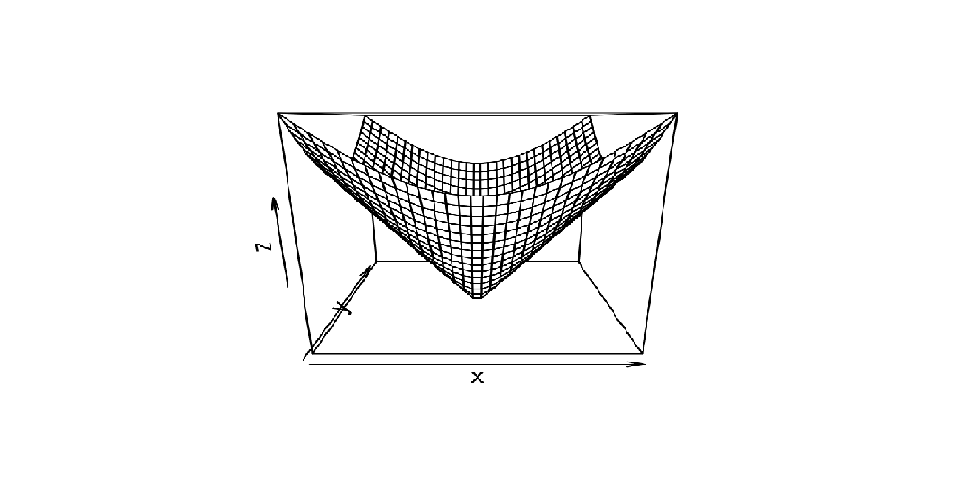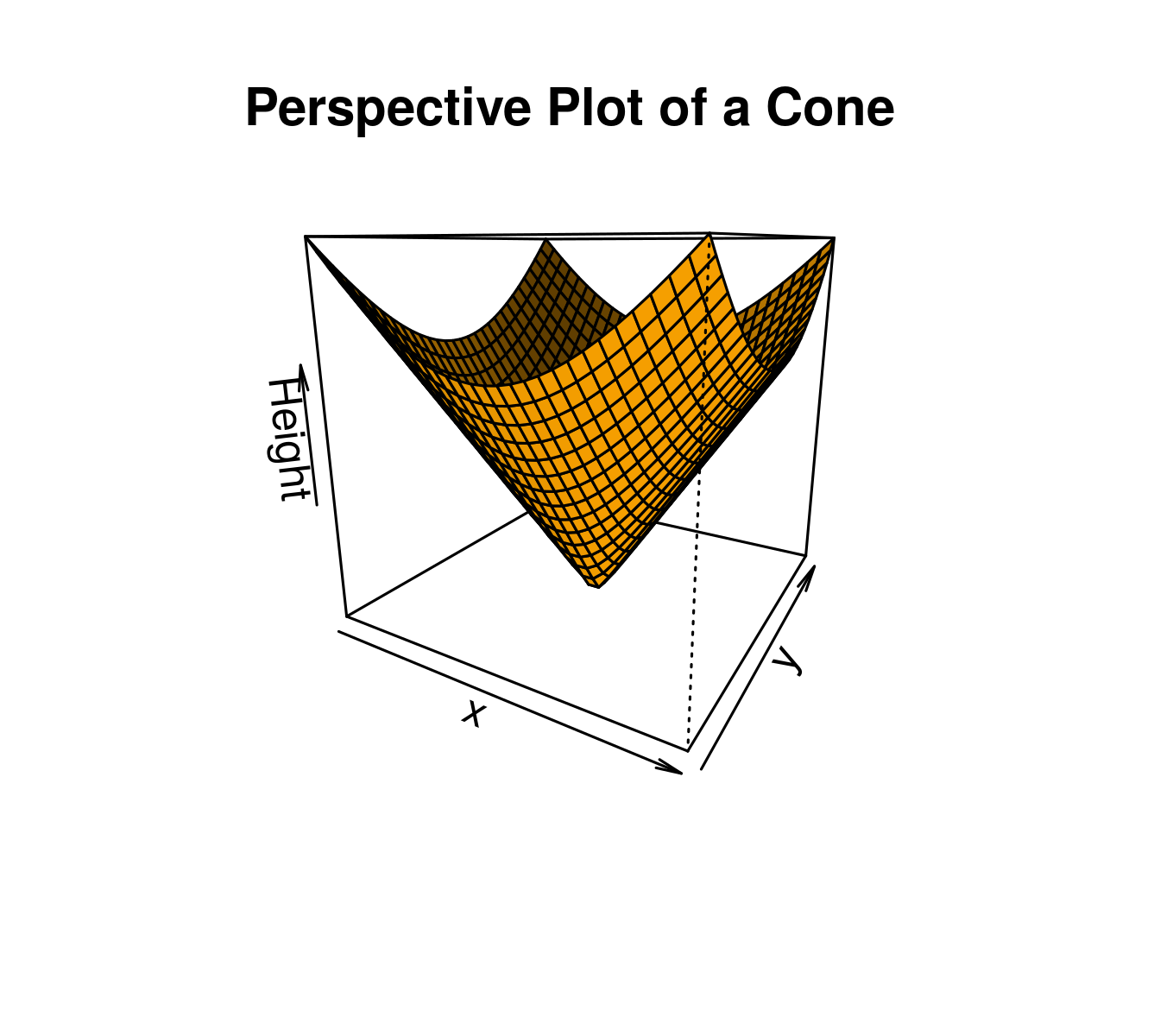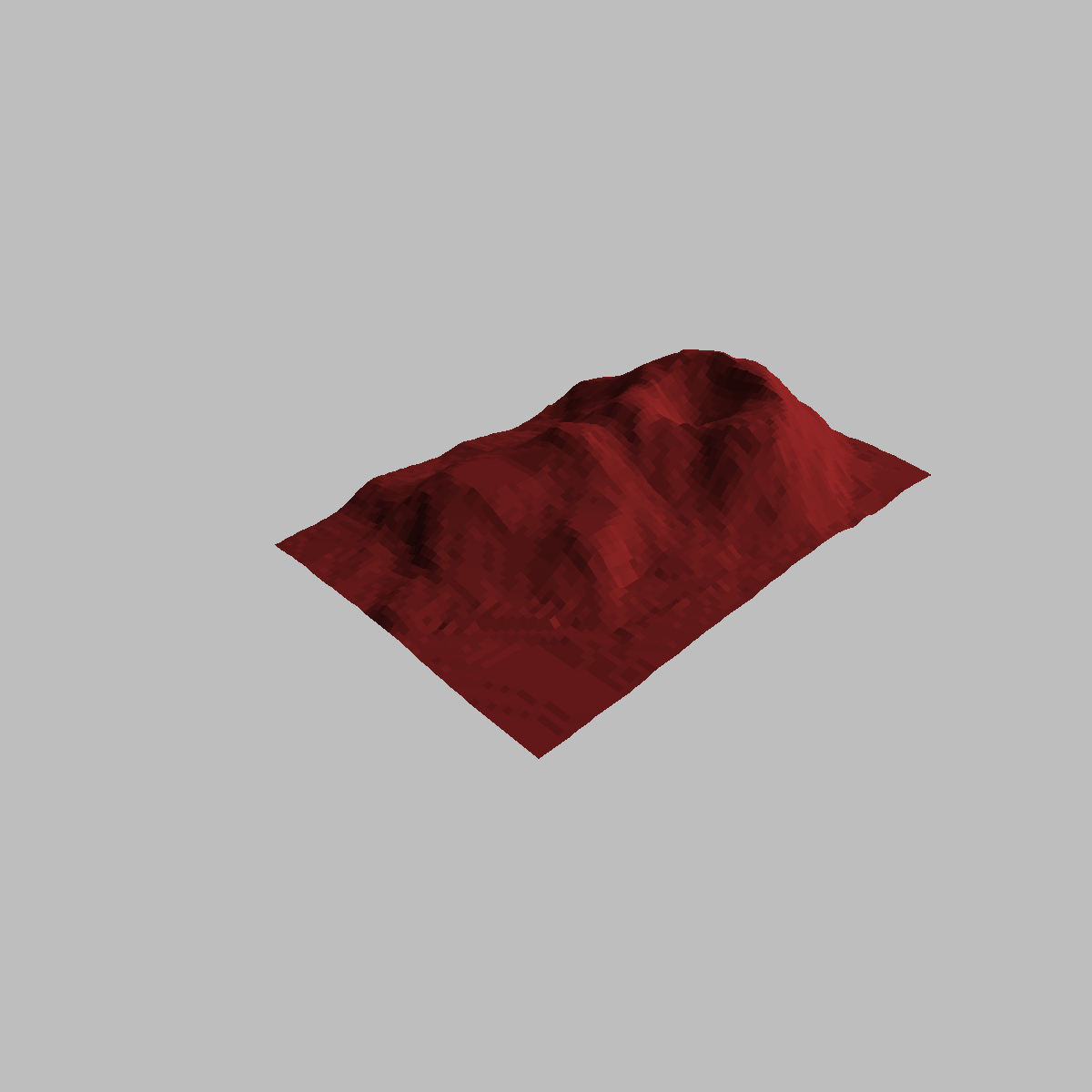# Creating 3D Plots in R Programming – persp() Function

3D plot in R Language is used to add title, change viewing direction, and add color and shade to the plot. The `persp()` function which is used to create 3D surfaces in perspective view. This function will draw perspective plots of a surface over the x–y plane. `persp()` is defines as a generic function. Moreover, it can be used to superimpose additional graphical elements on the 3D plot, by `lines()` or `points()`, using the function `trans3d()`.

Syntax: persp(x, y, z)

Parameter: This function accepts different parameters i.e. x, y and z where x and y are vectors defining the location along x- and y-axis. z-axis will be the height of the surface in the matrix z.

Return Value: persp() returns the viewing transformation matrix for projecting 3D coordinates (x, y, z) into the 2D plane using homogeneous 4D coordinates (x, y, z, t).

Example 1: Simple Right Circular Cone

 `# To illustrate simple right circular cone ` `cone <``-` `function(x, y){ ` `sqrt(x ^ ``2` `+` `y ^ ``2``) ` `} ` ` `  `# prepare variables. ` `x <``-` `y <``-` `seq(``-``1``, ``1``, length ``=` `30``) ` `z <``-` `outer(x, y, cone) ` ` `  `# plot the 3D surface ` `persp(x, y, z) `

Output:• Here in above code, function `seq()` to generate vector of equally spaced numbers.
• the `outer()` function to apply the function cone at every combination of x and y.

Example 2: Adding Titles and Labeling Axes to Plot

 `# Adding Titles and Labeling Axes to Plot ` `cone <``-` `function(x, y){ ` `sqrt(x ^ ``2` `+` `y ^ ``2``) ` `} ` ` `  `# prepare variables. ` `x <``-` `y <``-` `seq(``-``1``, ``1``, length ``=` `30``) ` `z <``-` `outer(x, y, cone) ` ` `  `# plot the 3D surface ` `# Adding Titles and Labeling Axes to Plot ` `persp(x, y, z, ` `main``=``"Perspective Plot of a Cone"``, ` `zlab ``=` `"Height"``, ` `theta ``=` `30``, phi ``=` `15``, ` `col ``=` `"orange"``, shade ``=` `0.4``) `

Output:• Here in above code, xlab, ylab and zlab can be used to label the three axes.
• theta and phi are viewing direction.

Example 3: Visualizing a simple DEM(Digital elevation model)

 `# Visualizing a simple DEM model ` ` `  `z <``-` `2` `*` `volcano        ``# Exaggerate the relief ` `x <``-` `10` `*` `(``1``:nrow(z))   ``# 10 meter spacing (S to N) ` `y <``-` `10` `*` `(``1``:ncol(z))   ``# 10 meter spacing (E to W) ` ` `  ` `  `# Don't draw the grid lines :  border = NA ` `par(bg ``=` `"gray"``) ` `persp(x, y, z, theta ``=` `135``, phi ``=` `30``, col ``=` `"brown"``, scale ``=` `FALSE, ` `      ``ltheta ``=` `-``120``, shade ``=` `0.75``, border ``=` `NA, box ``=` `FALSE) `

Output:My Personal Notes arrow_drop_upCheck out this Author's contributed articles.

If you like GeeksforGeeks and would like to contribute, you can also write an article using contribute.geeksforgeeks.org or mail your article to contribute@geeksforgeeks.org. See your article appearing on the GeeksforGeeks main page and help other Geeks.

Please Improve this article if you find anything incorrect by clicking on the "Improve Article" button below.

Article Tags :

1

Please write to us at contribute@geeksforgeeks.org to report any issue with the above content.